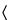785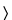OSMOLALITY AND OSMOLARITY

INTRODUCTION
Osmotic pressure plays a critical role in all biological processes that involve diffusion of solutes or transfer of fluids through membranes. Osmosis occurs when solvent but not solute molecules cross a semipermeable membrane from regions of lower to higher concentrations to produce equilibrium. The knowledge of osmotic pressures is important for practitioners in determining whether a parenteral solution is hypo-osmotic, iso-osmotic, or hyperosmotic. A quantitative measure of osmotic pressure facilitates the dilution required to render a solution iso-osmotic relative to whole blood.

OSMOTIC PRESSURE
The osmotic pressure of a solution depends on the number of particles in solution, and is therefore referred to as a colligative property. A particle can be a molecule or an ion or an aggregated species (e.g., a dimer) that can exist discretely in solution. A solution exhibits ideal behavior when no interaction occurs between solutes and solvent, except where solvent molecules are bound to solutes by hydrogen bonding or coordinate covalency. For such a solution containing a nondissociating solute, the osmotic pressure (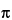) is directly proportional to its molality (number of moles of solute per kilogram of the solvent):= (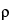RT/1000)m,
whereis the density of the solvent at the temperature T (in the absolute scale); R is the universal gas constant; and m is the molality of the solution. For a real solution containing more than one solute, the osmotic pressure is given by the formula:= (RT/1000)S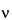imiFm,i
wherei is the number of particles formed by the dissociation of one molecule of the ith solute;i = 1 for nonionic (nondissociating) solutes; mi is the molality of the ith solute; and Fm,i is the molal osmotic coefficient of the ith solute. The molal osmotic coefficient takes into account the deviation of a solution from ideal behavior. Its value depends upon the concentration of the solute(s) in solution, its chemical properties, and ionic characteristics. The value of the molal osmotic coefficient of a solute can be determined experimentally by measuring the freezing point depression at different molal concentrations. At concentrations of pharmaceutical interest, the value of the molal osmotic coefficient is less than one. The molal osmotic coefficient decreases with the increase in concentration of the solute (Table 1).

OSMOLALITY
The osmolality of a solution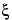m is given bym = SimiFm,i.
The osmolality of a real solution corresponds to the molality of an ideal solution containing nondissociating solutes and is expressed in osmoles or milliosmoles per kilogram of solvent (Osmol per kg or mOsmol per kg, respectively), a unit that is similar to the molality of the solution. Thus, osmolality is a measure of the osmotic pressure exerted by a real solution across a semipermeable membrane. Like osmotic pressure, other colligative properties of a solution, such as vapor pressure lowering, boiling point elevation, and freezing point depression, are also directly related to the osmolality of the solution. Indeed, the osmolality of a solution is typically determined most accurately and conveniently by measuring freezing point depression (DTf):
DTf = kfm
where kf is the molal cryoscopic constant, which is a property of the solvent. For water, the value of kf is 1.860per Osmol. That is, 1 Osmol of a solute added to 1 kg of water lowers the freezing point by 1.860.

OSMOLARITY
Osmolarity of a solution is a theoretical quantity expressed in osmoles per L (Osmol per L) of a solution and is widely used in clinical practice because it expresses osmoles as a function of volume. Osmolarity cannot be measured but is calculated theoretically from the experimentally measured value of osmolality.
Sometimes, osmolarity (c) is calculated theoretically from the molar concentrations:c = Sici
wherei is as defined above, and ci is the molar concentration of the ith solute in solution. For example, the osmolarity of a solution prepared by dissolving 1 g of vancomycin in 100 mL of 0.9% sodium chloride solution can be calculated as follows:
[3 × 10 g/L/1449.25(mol. wt. of vancomycin) + 2 × 9 g/L/58.44(mol. wt. of sodium chloride)] × 1000 = 329 mOsmol/L
The results suggest that the solution is slightly hyperosmotic because the osmolality of blood ranges between 285 and 310 mOsmol per kg. However, the solution is found to be hypo-osmotic and has an experimentally determined osmolality of 255 mOsmol per kg.1 The example illustrates that osmolarity values calculated theoretically from the concentration of a solution should be interpreted cautiously and may not represent the osmotic properties of infusion solutions.
The discrepancy between theoretical (osmolarity) and experimental (osmolality) results is, in part, due to the fact that osmotic pressure is related to osmolality and not osmolarity. More significantly, the discrepancy between experimental results and the theoretical calculation is due to the fact that the osmotic pressure of a real solution is less than that of an ideal solution because of interactions between solute molecules or between solute and solvent molecules in a solution. Such interactions reduce the pressure exerted by solute molecules on a semipermeable membrane, reducing experimental values of osmolality compared to theoretical values. This difference is related to the molal osmotic coefficient (Fm,i). The example also illustrates the importance of determining the osmolality of a solution experimentally, rather than calculating the value theoretically.

MEASUREMENT OF OSMOLALITY
The osmolality of a solution is commonly determined by the measurement of the freezing point depression of the solution.
Apparatus— The apparatus, an osmometer for freezing point depression measurement, consists of the following: a means of cooling the container used for the measurement; a resistor sensitive to temperature (thermistor), with an appropriate current- or potential-difference measurement device that may be graduated in temperature change or in osmolality; and a means of mixing the sample.
Osmometers that measure the vapor pressures of solutions are less frequently employed. They require a smaller volume of specimen (generally about 5 µL), but the accuracy and precision of the resulting osmolality determination are comparable to those obtained by the use of osmometers that depend upon the observed freezing points of solutions.
Standard Solutions— Prepare Standard Solutions as specified in Table 1, as necessary.2
Table 1. Standard Solutions for Osmometer Calibration*
Standard Solutions
(Weight in g of sodium chloride per kg of water)
Osmolality
(mOsmol/kg)
(m)
Molal Osmotic
Coefficient
(Fm, NaCl)
Freezing Point
Depression ()
DTf
3.087 100 0.9463 0.186
6.260 200 0.9337 0.372
9.463 300 0.9264 0.558
12.684 400 0.9215 0.744
15.916 500 0.9180 0.930
19.147 600 0.9157 1.116
22.380 700 0.9140 1.302
*  Adapted from the European Pharmacopoeia, 4th Edition, 2002, p. 50.
Test Solution— For a solid for injection, constitute with the appropriate diluent as specified in the instructions on the labeling. For solutions, use the sample as is. [note—A solution can be diluted to bring it within the range of measurement of the osmometer, if necessary, but the results must be expressed as that of the diluted solution and must NOT be multiplied by a dilution factor to calculate the osmolality of the original solution, unless otherwise indicated in the monograph. The molal osmotic coefficient is a function of concentration. Therefore, it changes with dilution. ]
Procedure— First, calibrate the instrument by the manufacturer's instructions. Confirm the instrument calibration with at least one solution from Table 1 such that the osmolality of the Standard Solution lies within 50 mOsmol/kg of the expected value of the Test Solution or the center of the expected range of osmolality of the Test Solution. The instrument reading should be within ±4 mOsmol per kg from the Standard Solution. Introduce an appropriate volume of each Standard Solution into the measurement cell as in the manufacturer's instructions, and start the cooling system. Usually, the mixing device is programmed to operate at a temperature below the lowest temperature expected from the freezing point depression. The apparatus indicates when the equilibrium is attained. If necessary, calibrate the osmometer, using an appropriate adjustment device such that the reading corresponds to either the osmolality or freezing point depression value of the Standard Solution shown in Table 1. [note—If the instrument reading indicates the freezing point depression, the osmolality can be derived by using the appropriate formula under Osmolality. ] Repeat the procedure with each Test Solution. Read the osmolality of the Test Solution directly, or calculate it from the measured freezing point depression.
Assuming that the value of the osmotic coefficient is essentially the same whether the concentration is expressed in molality or molarity, the experimentally determined osmolality of a solution can be converted to osmolarity in the same manner in which the concentration of a solution is converted from molality to molarity. Unless a solution is very concentrated, the osmolarity of a solution (c) can be calculated from its experimentally determined osmolality (m):c = 1000m / (1000 /+ Swii)
where wi is the weight in g; andi is the partial specific volume, in mL per g, of the ith solute. The partial specific volume of a solute is the change in volume of a solution when an additional 1 g of solute is dissolved in the solution. This volume can be determined by the measurement of densities of the solution before and after the addition of the solute. The partial specific volumes of salts are generally very small, around 0.1 mL per g. However, those of other solutes are generally higher. For example, the partial specific volumes of amino acids are in the range of 0.6–0.9 mL per g. It can be shown from the above equation correlating osmolarity with osmolality that,c =m (c)
whereis the density of the solution, and c is the total solute concentration, both expressed in g per mL. Thus, alternatively, the osmolarity can also be calculated from experimentally determined osmolality from the measurement of density of the solution by a suitable method and the total weight of the solute, after correction for water content, dissolved per mL of the solution.

1  Kastango, E.S. and Hadaway, L. International Journal of Pharmaceutical Compounding 5, (2001) 465-469.
2  Commercially available solutions for osmometer calibration, with osmolalities equal to or different from those listed in Table 1 and standardized by methods traceable to NIST, may be used.
Auxiliary Information— Please check for your question in the FAQs before contacting USP.
Topic/Question Contact Expert Committee
General Chapter Horacio N. Pappa, Ph.D.
Principal Scientific Liaison
1-301-816-8319
(GCPA2010) General Chapters - Physical Analysis
USP35–NF30 Page 335
Pharmacopeial Forum: Volume No. 34(5) Page 1251